# In regression, we assume noise is independent of all measured predictors. What happens if it isn't?

A number of key assumptions underlie the linear regression model - among them linearity and normally distributed noise (error) terms with constant variance In this post, I consider an additional assumption: the unobserved noise is uncorrelated with any covariates or predictors in the model.

In this simple model:

$Y_i = \beta_0 + \beta_1X_i + e_i,$

$$Y_i$$ has both a structural and stochastic (random) component. The structural component is the linear relationship of $$Y$$ with $$X$$. The random element is often called the $$error$$ term, but I prefer to think of it as $$noise$$. $$e_i$$ is not measuring something that has gone awry, but rather it is variation emanating from some unknown, unmeasurable source or sources for each individual $$i$$. It represents everything we haven’t been able to measure.

Our goal is to estimate $$\beta_1$$, which characterizes the structural linear relationship of $$X$$ and $$Y$$. When we estimate the model, we get a quantity $$\hat{\beta_1}$$, and we hope that on average we do pretty well (i.e. if we were to estimate $$\beta_1$$ repeatedly, $$E[\hat{\beta_1}] = \beta_1$$). In order for us to make sure that is the case, we need to assume that $$e_i$$ and $$X_i$$ are independent. In other words, the sources that comprise $$e_i$$ must not be related in way to whatever it is that $$X_i$$ is measuring.

### Correlation without causation

First, I’ll generate $$X's$$ and $$e's$$ that are correlated with a using a data generation process that makes no assumptions about the underlying causal process. The provides a picture of how $$\hat{\beta_1}$$ might diverge from the true $$\beta_1$$.

library(simstudy)
set.seed(3222)

dT <- genCorData(500, mu = c(0, 0), sigma = c(sqrt(1.25), 1),
rho = 0.446, corstr = "cs", cnames = c("X","eCor"))

Outcome $$Y$$ is based on $$X$$ and $$e_{cor}$$. For comparison’s sake, I generate a parallel outcome that is also based on $$X$$ but the noise variable $$e_{ind}$$ is independent of $$X$$:

def <- defDataAdd(varname = "Ycor", formula = "X + eCor",
dist = "nonrandom")
def <- defDataAdd(def, varname = "eInd", formula = 0, variance = 1,
dist = "normal" )
def <- defDataAdd(def, varname = "Yind", formula = "X + eInd",
dist = "nonrandom")

dT
##       id          X       eCor       Ycor       eInd        Yind
##   1:   1 -1.1955846 -0.1102777 -1.3058624  1.1369435 -0.05864113
##   2:   2 -0.4056655 -0.6709221 -1.0765875 -0.8441431 -1.24980856
##   3:   3 -0.5893938  1.2146488  0.6252550 -0.2666314 -0.85602516
##   4:   4  0.9090881  0.3108645  1.2199526  0.3397857  1.24887377
##   5:   5 -2.6139989 -1.7382986 -4.3522975 -0.1793858 -2.79338470
##  ---
## 496: 496  3.1615624  0.6160661  3.7776285  0.4658992  3.62746167
## 497: 497  0.6416140  0.1031316  0.7447456 -0.1440062  0.49760784
## 498: 498  0.1340967 -0.4029388 -0.2688421  0.6165793  0.75067604
## 499: 499 -1.2381040  0.8197002 -0.4184038  0.6717294 -0.56637463
## 500: 500 -0.7159373 -0.0905287 -0.8064660  0.9148175  0.19888019

The observed $$X$$ and $$e_{cor}$$ are correlated, but $$X$$ and $$e_{ind}$$ are not:

dT[, cor(cbind(X, eCor))]
##              X      eCor
## X    1.0000000 0.4785528
## eCor 0.4785528 1.0000000
dT[, cor(cbind(X, eInd))]
##                X        eInd
## X     1.00000000 -0.02346812
## eInd -0.02346812  1.00000000

On the left below is a plot of outcome $$Y_{ind}$$ as a function of $$X$$. The red line is the true structural component defining the relationship between these two variables. The points are scattered around that line without any clear pattern, which is indicative of independent noise.

The plot on the right shows $$Y_{cor}$$ as a function of $$X$$. Since the stochastic component of $$Y_{cor}$$ is the correlated noise, the lower $$X$$ values are more likely to fall below the true line, and the larger $$X$$ values above. The red line does not appear to be a very good fit in this case; this is the bias induced by correlated noise.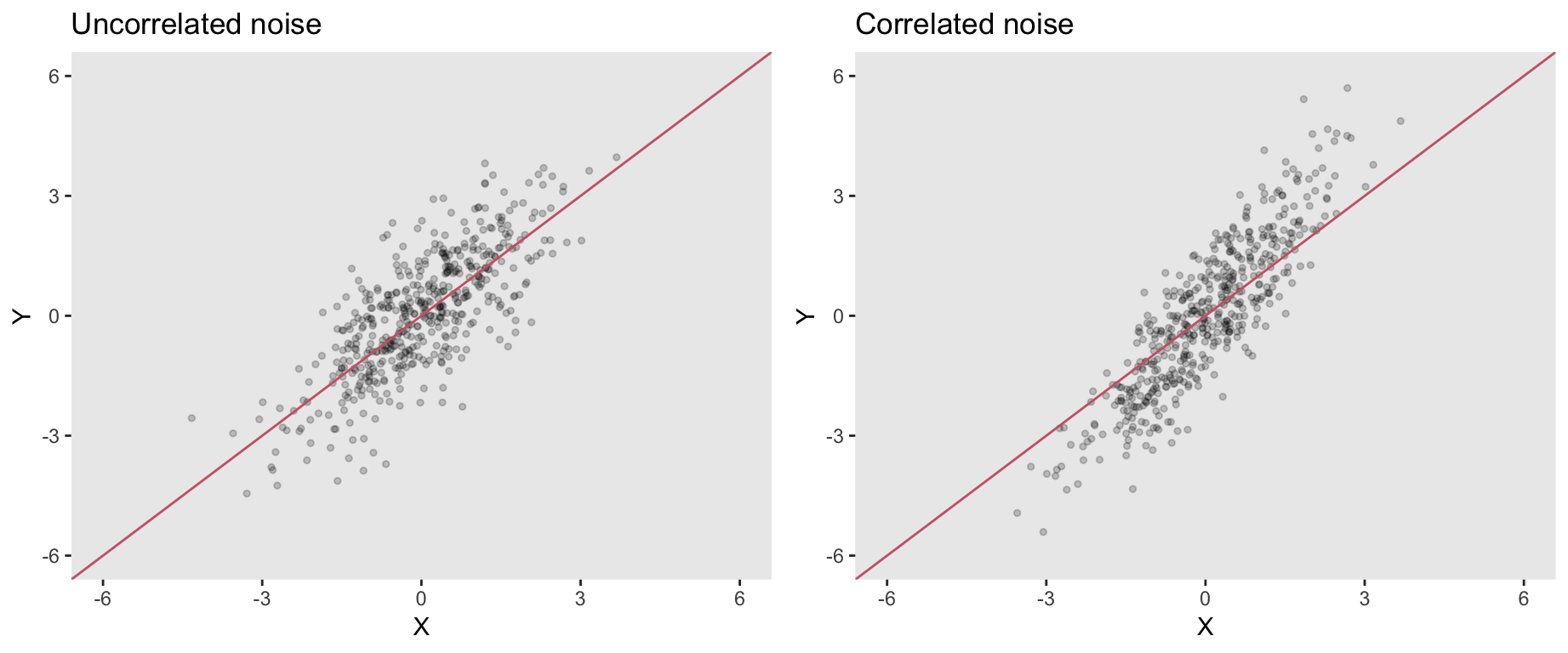The model fits corroborate the visual inspection. $$\hat{\beta_1}$$ based on uncorrelated noise is close to 1, the true value:

fit2 <- lm(Yind ~ X, data = dT)
rndTidy(fit2)
##           term estimate std.error statistic p.value
## 1: (Intercept)     0.06      0.05      1.37    0.17
## 2:           X     0.98      0.04     25.75    0.00

$$\hat{\beta_1}$$ based on correlated noise is 1.42, larger than the true value:

fit1 <- lm(Ycor ~ X, data = dT)
rndTidy(fit1)
##           term estimate std.error statistic p.value
## 1: (Intercept)    -0.01      0.04     -0.25     0.8
## 2:           X     1.42      0.03     41.28     0.0

A plot of the fitted (blue) line based on the biased estimate clearly shows the problem of regression estimation in this context: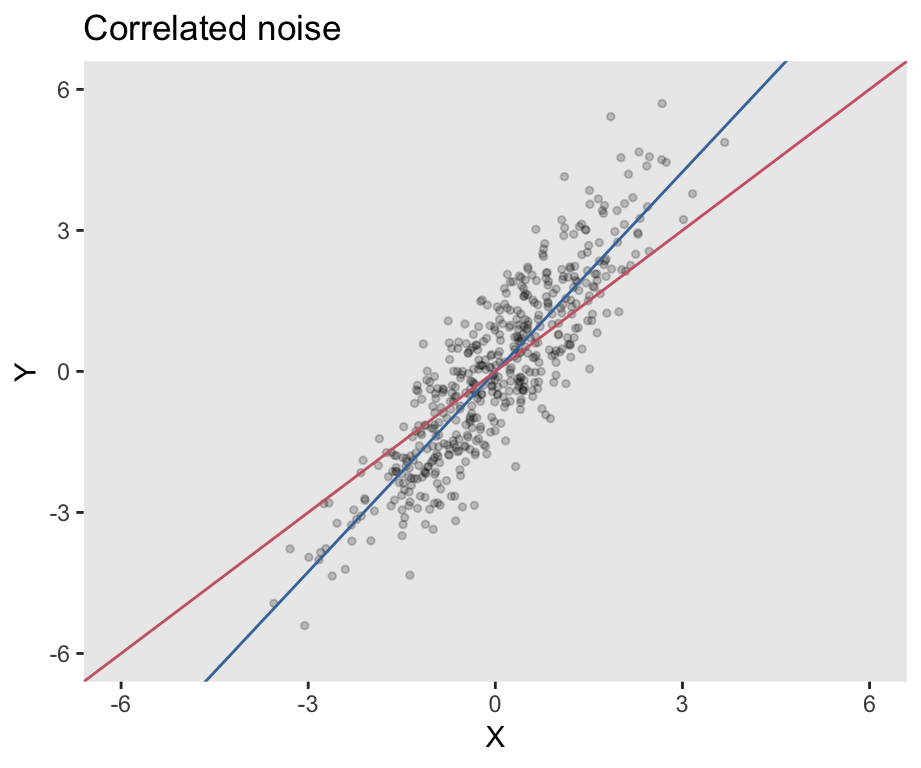### Thinking about underlying causality and noise

Here is a pure thought exercise to consider this bias induced by the correlation. Fundamentally, the implications depend on the purpose of the model. If we are using the model for description or prediction, we may not care about the bias. For example, if we are describing how $$Y$$ changes as $$X$$ changes in some population, the underlying data generation process may not be of interest. Likewise, if our goal is predicting $$Y$$ based on an observed $$X$$, the biased estimate of $$\beta_1$$ may be adequate.

However, if we are interested in understanding how intervening or changing the level of $$X$$ at the individual level effects the outcome $$Y$$ for that individual, then an unbiased estimate of $$\beta_1$$ becomes more important, and noise that is correlated with the predictor of interest could be problematic.

However, in a causal context, all noise may not be created equally. Consider these two different causal models: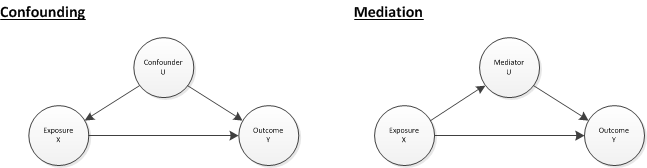We can generate identically distributed data based on these two mechanisms:

# Confounding

defc <- defData(varname = "U", formula=0, variance=1, dist="normal")
defc <- defData(defc, "X", "0.5*U", 1, "normal")
defc <- defData(defc, "Y", "X + U", dist = "nonrandom")

dcon <- genData(1000, defc)
# Mediation

defm <- defData(varname="X", formula=0, variance =1.25, dist="normal")
defm <- defData(defm, "U", ".4*X", .8, "normal")
defm <- defData(defm, "Y", "X + U", dist = "nonrandom")

dmed <- genData(1000, defm)

The observed covariance between $$X$$ and $$U$$ (the noise) is similar for the two processes …

dcon[, var(cbind(X,U))]
##           X         U
## X 1.2516199 0.5807696
## U 0.5807696 1.0805321
dmed[, var(cbind(X,U))]
##           X         U
## X 1.2365285 0.5401577
## U 0.5401577 1.0695366

… as is the model fit for each: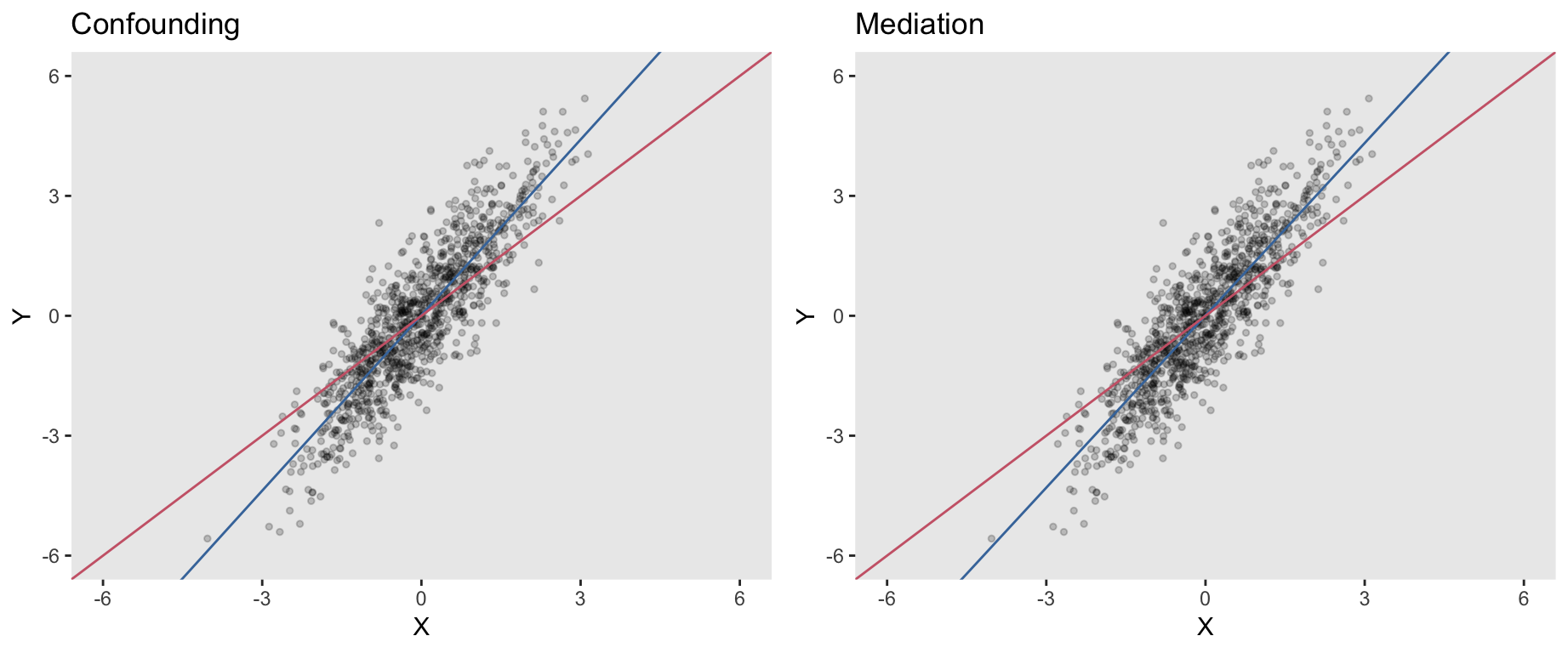And here is a pair of histograms of estimated values of $$\beta_1$$ for each data generating process, based on 1000 replications of samples of 100 individuals. Again, pretty similar: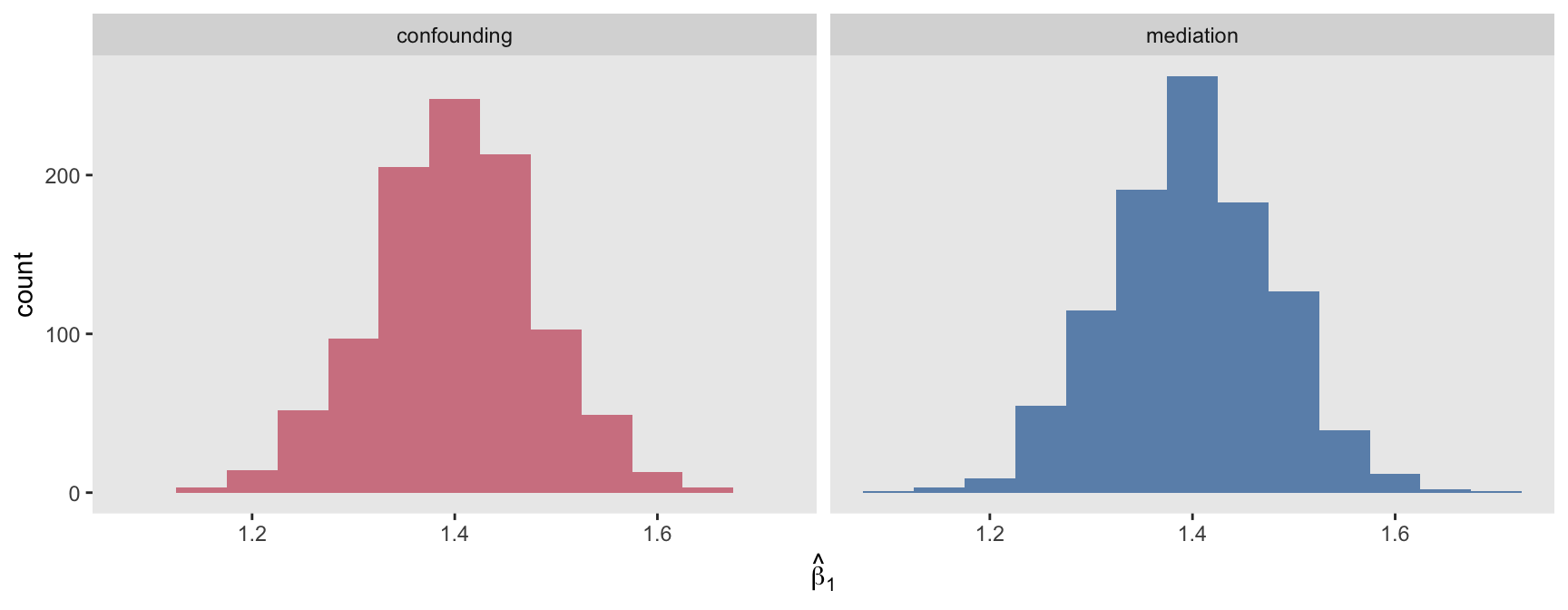Despite the apparent identical nature of the two data generating processes, I would argue that biased estimation is only a problem in the context of confounding noise. If we intervene on $$X$$ without changing $$U$$, which could occur in the context of unmeasured confounding, the causal effect of $$X$$ on $$Y$$ would be overestimated by the regression model. However, if we intervene on $$X$$ in the context of a process that involves mediation, it would be appropriate to consider all the post-intervention effects of changing $$X$$, so the “biased” estimate may in fact be the appropriate one.

The key here, of course, is that we cannot verify this unobserved process. By definition, the noise is unobservable and stochastic. But, if we are developing models that involve causal relations of unmeasured quantities, we have to be explicit about the causal nature underlying these unmeasured quantities. That way, we know if we should be concerned about hidden correlation or not.